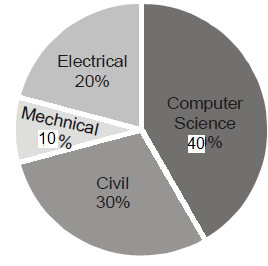Open in App
Not now

# GATE | GATE-CS-2015 (Set 1) | Question 9

• Last Updated : 28 Jun, 2021

The pie chart below has the breakup of the number of students, from different departments in an engineering college for the year 2012. The proportion of male to female students in each department is 5 : 4. There are 40 males in Electrical Engineering. What is the different between the numbers of female students in the Civil department and the female students in the Mechanical department?(A) 32
(B) 16
(C) 48
(D) 8

Explanation: Total male students in Electrical = 40
Total female in Electrical = (40 * 4)/5 = 32

Total female in Mechanical = 32/2 = 16
Total female in Civil = [(30 + 42)*3/2]*4/9 = = 72 * 2/3 = 48

The difference is 32.

Quiz of this Question

My Personal Notes arrow_drop_up
Related Articles